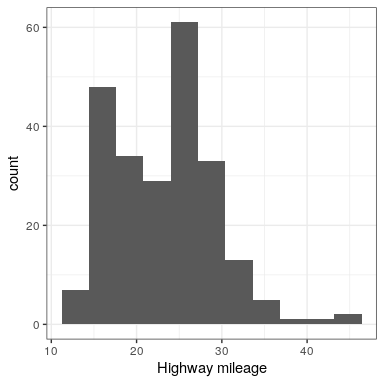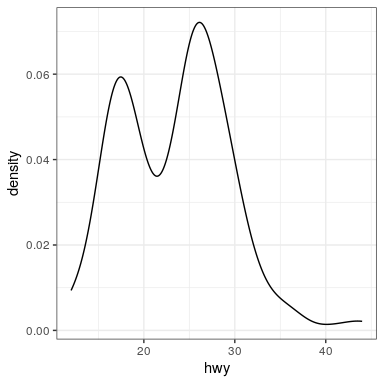# 7.4: Plotting the Distribution of a Single Variable

•• Russell A. Poldrack & Anna Khazenzon
• Stanford University
$$\newcommand{\vecs}{\overset { \rightharpoonup} {\mathbf{#1}} }$$ $$\newcommand{\vecd}{\overset{-\!-\!\rightharpoonup}{\vphantom{a}\smash {#1}}}$$$$\newcommand{\id}{\mathrm{id}}$$ $$\newcommand{\Span}{\mathrm{span}}$$ $$\newcommand{\kernel}{\mathrm{null}\,}$$ $$\newcommand{\range}{\mathrm{range}\,}$$ $$\newcommand{\RealPart}{\mathrm{Re}}$$ $$\newcommand{\ImaginaryPart}{\mathrm{Im}}$$ $$\newcommand{\Argument}{\mathrm{Arg}}$$ $$\newcommand{\norm}{\| #1 \|}$$ $$\newcommand{\inner}{\langle #1, #2 \rangle}$$ $$\newcommand{\Span}{\mathrm{span}}$$ $$\newcommand{\id}{\mathrm{id}}$$ $$\newcommand{\Span}{\mathrm{span}}$$ $$\newcommand{\kernel}{\mathrm{null}\,}$$ $$\newcommand{\range}{\mathrm{range}\,}$$ $$\newcommand{\RealPart}{\mathrm{Re}}$$ $$\newcommand{\ImaginaryPart}{\mathrm{Im}}$$ $$\newcommand{\Argument}{\mathrm{Arg}}$$ $$\newcommand{\norm}{\| #1 \|}$$ $$\newcommand{\inner}{\langle #1, #2 \rangle}$$ $$\newcommand{\Span}{\mathrm{span}}$$$$\newcommand{\AA}{\unicode[.8,0]{x212B}}$$

How do you choose which geometry to use? ggplot allows you to choose from a number of geometries. This choice will determine what sort of plot you create. We will use the built-in mpg dataset, which contains fuel efficiency data for a number of different cars.

## 7.4.1 Histogram

The histogram shows the ogerall distribution of the data. Here we use the nclass.FD function to compute the optimal bin size.

ggplot(mpg, aes(hwy)) +
geom_histogram(bins = nclass.FD(mpg\$hwy)) +
xlab('Highway mileage')Instead of creating discrete bins, we can look at relative density continuously.

## 7.4.2 Density plot

ggplot(mpg, aes(hwy)) +
geom_density() +
xlab('Highway mileage')A note on defaults: The default statistic (or “stat”) underlying geom_density is called “density” – not surprising. The default stat for geom_histogram is “count”. What do you think would happen if you overrode the default and set stat="count"?

ggplot(mpg, aes(hwy)) +
geom_density(stat = "count")

What we discover is that the geometric difference between geom_histogram and geom_density can actually be generalized. geom_histogram is a shortcut for working with geom_bar, and geom_density is a shortcut for working with geom_line.

## 7.4.3 Bar vs. line plots

ggplot(mpg, aes(hwy)) +
geom_bar(stat = "count")Note that the geometry tells ggplot what kind of plot to use, and the statistic (stat) tells it what kind of summary to present.

ggplot(mpg, aes(hwy)) +
geom_line(stat = "density")This page titled 7.4: Plotting the Distribution of a Single Variable is shared under a not declared license and was authored, remixed, and/or curated by Russell A. Poldrack & Anna Khazenzon via source content that was edited to the style and standards of the LibreTexts platform; a detailed edit history is available upon request.